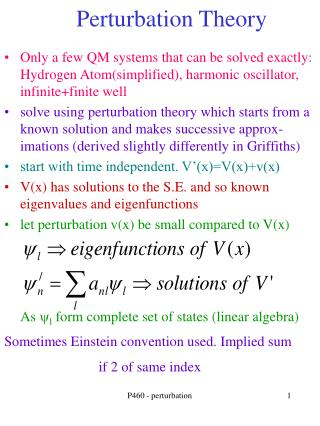DownloadDownload PresentationPerturbation Theory

# Perturbation Theory

Download Presentation## Perturbation Theory

- - - - - - - - - - - - - - - - - - - - - - - - - - - E N D - - - - - - - - - - - - - - - - - - - - - - - - - - -
##### Presentation Transcript

1. Perturbation Theory • Only a few QM systems that can be solved exactly: Hydrogen Atom(simplified), harmonic oscillator, infinite+finite well • solve using perturbation theory which starts from a known solution and makes successive approx- imations (derived slightly differently in Griffiths) • start with time independent. V’(x)=V(x)+v(x) • V(x) has solutions to the S.E. and so known eigenvalues and eigenfunctions • let perturbation v(x) be small compared to V(x) As yl form complete set of states (linear algebra) Sometimes Einstein convention used. Implied sum if 2 of same index P460 - perturbation

2. Plug into Schrod. Eq. • know solutions for V • use orthogonality • multiply each side by wave function* and integrate • matrix element of potential v is defined: P460 - perturbation

3. One solution: assume perturbed wave function very close to unperturbed (matrix is unitary as “size” of wavefunction doesn’t change) • assume last term small. Take m=n. Energy difference is expectation value of perturbing potential **** P460 - perturbation

4. Time independent example • know eigenfunctions/values of infinite well. • Assume mostly in ground state n=1 P460 - perturbation

5. Time independent example • Get first order correction to wavefunction • only even Parity terms remain (rest identically 0) as • gives Even Parity P460 - perturbation

6. Time Dependent Perturbation Theory • Many possible potentials. Consider one where • V’(x,t)=V(x)+v(x,t) • V(x) has solutions to the S.E. and so known eigenvalues and eigenfunctions • let perturbation v(x,t) be small compared to V(x) • examples:finite square well plus a pulse or atoms in an oscillating electric field Solve Schrodinger.Eqn P460 - perturbation

7. Time Dependent • First line (in brackets[]) is equal to 0 • take “dot product” of other two terms with wave function Wave functions are orthogonal P460 - perturbation

8. Time Dependent :Example • This is exact. How to solve depend on the initial conditions and perturbation • take v(x,t)=0 t<0 and =v(x) t>0. Also assume all in k-state at t=0 and perturbation is small Probability to be in state m depends on the square of amplitude a. Only large when energies are ~same P460 - perturbation

9. Time Dependent :Example • The probability for state k to make a transition into any other state is: • evaluate P by assuming closely spaced states and replacing sum with integral Seen in Plank distribution. We see again later P460 - perturbation

10. Fermi Golden Rule • Assume first 2 terms vary slowly.Pull out of integral and evaluate the integral at the pole • this doesn’t always hold--ionization has large dE offset by larger density • n are states near k. Conserve energy. Rate depends on both the matrix element (which includes “physics”) and the density of states. Examples later on, especially in 461 P460 - perturbation